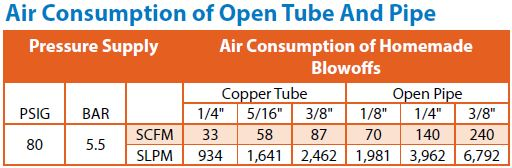## Estimating the Total Cost of Compressed AirIt is important to know the cost of compressed air at your facility.  Most people think that compressed air is free, but it is most certainly not.  Because of the expense, compressed air is considered to be a fourth utility in manufacturing plants.  In this blog, I will show you how to calculate the cost to make compressed air.  Then you can use this information to determine the need for Intelligent Compressed Air® products.

There are two types of air compressors, positive displacement and dynamic.  The core construction for both is an electric motor that spins a shaft.  Positive displacement types use the energy from the motor and the shaft to change the volume in an area, like a piston in a reciprocating compressor or like rotors in a rotary compressor.  The dynamic types use the energy from the motor and the shaft to create a velocity energy with an impeller.  (You can read more about air compressors HERE).  For electric motors, the power is described either in kilowatts (KW) or horsepower (hp).  As a unit of conversion, there are 0.746 KW in 1 hp.  The electric companies charge at a rate of kilowatt-hour (KWh).  So, we can determine the energy cost to spin the electric motors.  If your air compressor has a unit of horsepower, or hp, you can use Equation 1:

Equation 1:

hp * 0.746 * hours * rate / (motor efficiency)

where:

hp – horsepower of motor

0.746 – conversion to KW

hours – running time

rate – cost for electricity, KWh

motor efficiency – average for an electric motor is 95%.

If the air compressor motor is rated in kilowatts, or KW, then the above equation can become a little simpler, as seen in Equation 2:

Equation 2:

KW * hours * rate / (motor efficiency)

where:

KW – Kilowatts of motor

hours – running time

rate – cost for electricity, KWh

motor efficiency – average for an electric motor is 95%.

As an example, a manufacturing plant operates 250 day a year with 8-hour shifts.  The cycle time for the air compressor is roughly 50% on and off.  To calculate the hours of running time, we have 250 days at 8 hours/day with a 50% duty cycle, or 250 * 8 * 0.50 = 1,000 hours of running per year.  The air compressor that they have is a 100 hp rotary screw.  The electrical rate for this facility is at \$0.08/KWh. With these factors, the annual cost can be calculated by Equation 1:

100hp * 0.746 KW/hp * 1,000hr * \$0.08/KWh / 0.95 = \$6,282 per year.

In both equations, you can substitute your information to see what you actually pay to make compressed air each year at your facility.

The type of air compressor can help in the amount of compressed air that can be produced by the electric motor.  Generally, the production rate can be expressed in different ways, but I like to use cubic feet per minute per horsepower, or CFM/hp.

The positive displacement types have different values depending on how efficient the design.  For a single-acting piston type air compressor, the amount of air is between 3.1 to 3.3 CFM/hp.  So, if you have a 10 hp single-acting piston, you can produce between 31 to 33 CFM of compressed air.  For a 10 hp double-acting piston type, it can produce roughly 4.7 to 5.0 CFM/hp.  As you can see, the double-acting air compressor can produce more compressed air at the same horsepower.

The rotary screws are roughly 3.4 to 4.1 CFM/hp.  While the dynamic type of air compressor is roughly 3.7 – 4.7 CFM/hr.  If you know the type of air compressor that you have, you can calculate the amount of compressed air that you can produce per horsepower.  As an average, EXAIR uses 4 CFM/hp of air compressor when speaking with customers who would like to know the general output of their compressor.

With this information, we can estimate the total cost to make compressed air as shown in Equation 3:

Equation 3:

C = 1000 * Rate * 0.746 / (PR * 60)

where:

C – Cost of compressed air (\$ per 1000 cubic feet)

1000 – Scalar

Rate – cost of electricity (KWh)

0.746 – conversion hp to KW

PR – Production Rate (CFM/hp)

60 – conversion from minutes to hour

So, if we look at the average of 4 CFM/hp and an average electrical rate of \$0.08/KWh, we can use Equation 3 to determine the average cost to make 1000 cubic feet of air.

C = 1000 * \$0.08/KWh * 0.746 / (4 CFM/hp * 60) = \$0.25/1000ft3.

Once you have established a cost for compressed air, then you can determine which areas to start saving money.  One of the worst culprits for inefficient air use is open pipe blow-offs.  This would include cheap air guns, drilled holes in pipes, and tubes.  These are very inefficient for compressed air and can cost you a lot of money.  I will share a comparison to a 1/8” NPT pipe to an EXAIR Mini Super Air Nozzle.  (Reference below).  As you can see, by just adding the EXAIR nozzle to the end of the pipe, the company was able to save \$1,872 per year.  That is some real savings.